# What is Two Pointer Approach?

### Introduction:

This is one of the approaches which can be used to solve a problem in optimal time. First, we’ll understand every aspect of this approach and would later discuss a very common problem for a better understanding.

### What is the two pointer approach?

As the name suggests, a two-pointer approach uses two-pointers to find the answer to a problem in the optimal time. When the given data is present in a linear form i.e. in terms of an array, vector, linked list, etc we can use this approach.

This approach is very useful when you want to reduce the space complexity to constant but the state of time complexity still remains arbitrary i.e. it would reduce to a large extent or to a small extent.

An extra data structure can solve some problems with better time complexity but space complexity is increased which is a problem that could be addressed using this approach.

### Usage:

Two pointer approach can be used when you want to process two elements in a single iteration or determine a type of pattern.

Example: Detecting loop in a linked list, checking palindrome in arrays, strings, and linked list, reversing a string or a linked list, two sum problem, finding the middle of a linked list, etc.

### Assignment:

These pointers are generally assigned to the first element itself, or sometimes to the first element and the last element. The following diagrams explain the above statements.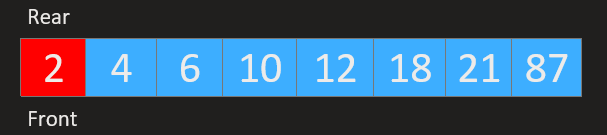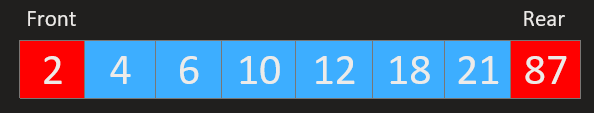For linked lists, we use the tortoise-harpe approach or the slow-fast pointer approach which is the same as two pointer approach.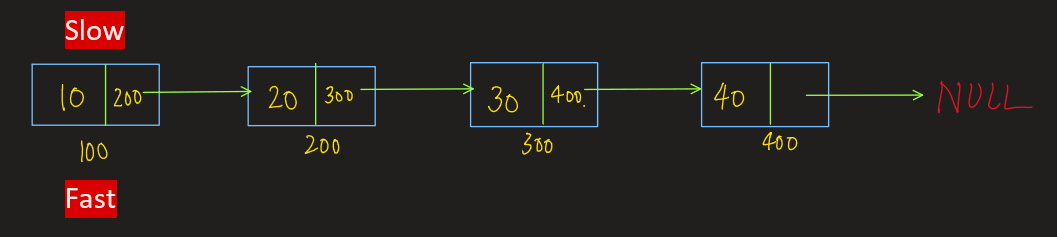Example

Let’s solve two problems: two sums and finding the middle element in the linked list to understand this approach in a better way.

Two Sum

Problem Statement: Given an array, find the indexes of two elements whose sum is equal to the given sum. If there exist multiple solutions, print the minimum indices.

Here we are assuming the array is sorted.

Examples:

```Example 1:

Input: arr[]={1,3,4,5,7,10,11,19,20}, sum=7
Output: {1,2}

Example 2:
Input: arr[]={2,9,13,21,54}, sum=63
Output: {1,4}
```

Approach

### Approach 1: Brute Force

We use a pointer i which would point to the first index of the array and another pointer j which would traverse across the array. Later we check if the sum of elements at indices i and j is equal to the sum. If it’s equal, we print i and j else we increment the i pointer and for every i we traverse the array using j.

Code:

## C++ Code

``````void twosum_brute(int arr[], int sum, int sizeArr) {
for (int i = 0; i < sizeArr - 1; i++) {
for (int j = i + 1; j < sizeArr; j++) {
if (arr[i] + arr[j] == sum) {
cout << i << " " << j << "\n";
return;
}
}
}
cout << "-1 -1" << "\n"; // if not found, print -1 -1
}
``````

Time complexity: O(n^2), since for every element we are traversing the whole array and there are n elements in the array.

Space complexity: O(1).

## Java Code

``````static void twosum_brute(int arr[], int sum, int sizeArr) {
for (int i = 0; i < sizeArr - 1; i++) {
for (int j = 0; j < sizeArr; j++) {
if (arr[i] + arr[j] == sum) {
System.out.println(i + " " + j);
return;
}
}
}
System.out.println("-1 -1");
}
``````

Time complexity: O(n^2), since for every element we are traversing the whole array and there are n elements in the array.

Space complexity: O(1).

### Approach 2: Two pointer approach

As the name suggests, we use two pointers low and high but the key is we perform a single iteration of the array. We mark low as 0 [beginning of the array] and high as size-1 [end of the array].

We calculate arr[low]+arr[high] and check:

1. If arr[low]+arr[high] < sum, we increment the low pointer.
2. If arr[low]+arr[high] = sum, we print low and high.
3. If arr[low]+arr[high] > sum, we decrement the high pointer.

The following diagram explains the approach.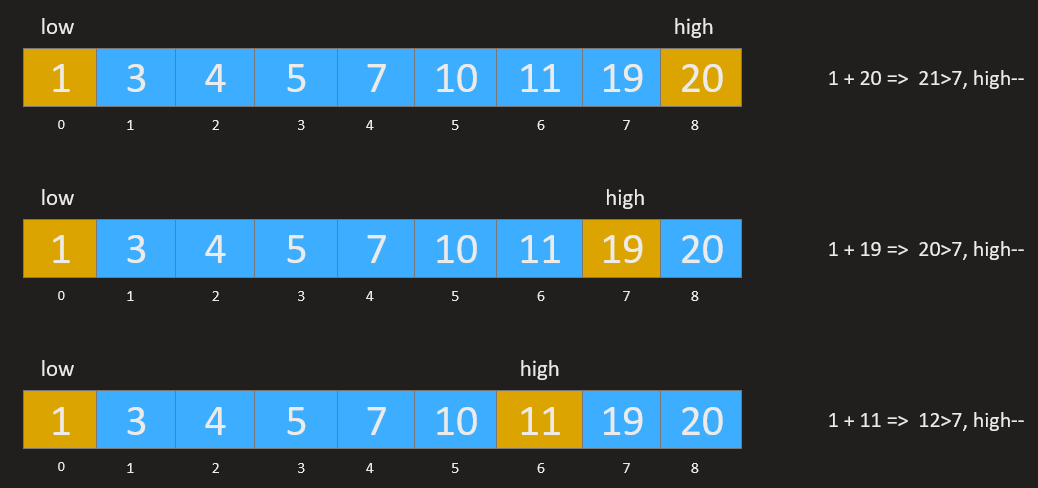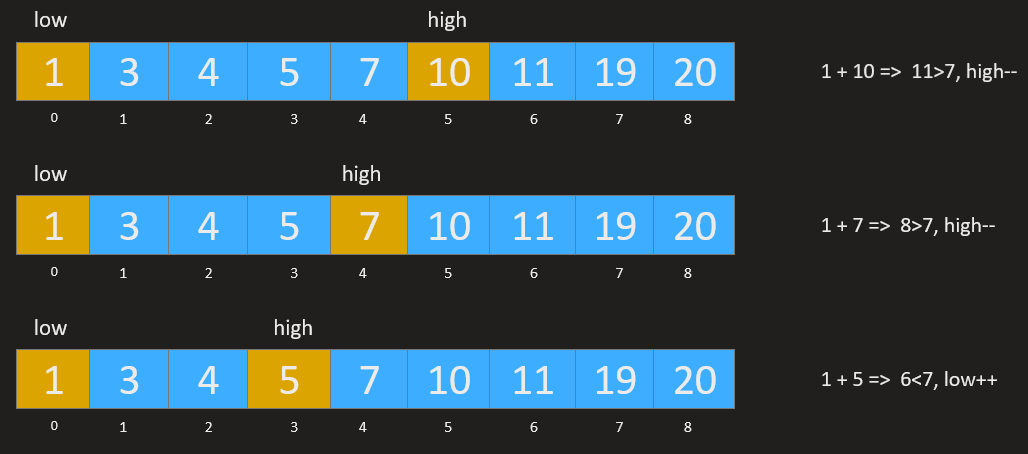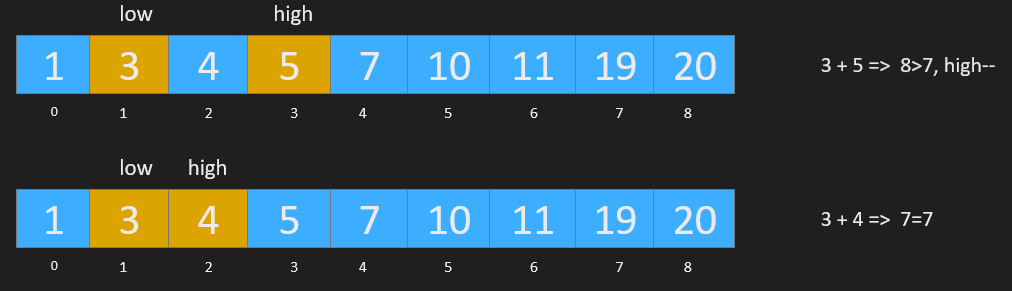Code:

## C++ Code

``````void twosum_pointer(int arr[], int sum, int sizeArr) {
int low = 0;
int high = sizeArr = sizeArr - 1;
while (low < high) {
if (arr[low] + arr[high] == sum) {
cout << low << " " << high << "\n";
return;
} else if (arr[low] + arr[high] < sum) low++;
else high--;
}
coût<<"-1 -1"<<"\n";
}
``````

Since we considered the array is sorted, the time complexity is reduced to O(n) and the space complexity reduces to O(1) unlike hashing where we were using maps and hashmaps to store elements and compare them.

## Java Code

``````static void twosum_pointer(int arr[], int sum) {
int low = 0;
int high = arr.length - 1;
while (low < high) {
if (arr[low] + arr[high] == sum) {
System.out.println(low + " " + high);
return;
} else if (arr[low] + arr[high] < sum) low++;
else high--;
}
System.out.println("-1 -1");
}
``````

Since we considered the array is sorted, the time complexity is reduced to O(n) and the space complexity reduces to O(1) unlike hashing where we were using maps and hashmaps to store elements and compare them.

Considering the array to be unsorted – firstly, we need to sort the array as the two pointer approach won’t work on unsorted arrays. This would increase time complexity to O(nlogn) which is still better than the brute force approach and better than hashing in terms of space used.

Check out these problems for a better understanding: palindrome or not in a linked list, Detect a cycle in a linked list.

Special thanks to Yash Mishra for contributing to this article on takeUforward. If you also wish to share your knowledge with the takeUforward fam, please check out this article